# Bihar Board Class 9th Maths Solutions Chapter 9 Areas of Parallelograms and Triangles Ex 9.4

Bihar Board Class 9th Maths Solutions Chapter 9 Areas of Parallelograms and Triangles Ex 9.4 Textbook Questions and Answers.

## BSEB Bihar Board Class 9th Maths Solutions Chapter 9 Areas of Parallelograms and Triangles Ex 9.4Question 1.
Parallelogram ABCD and rectangle ABEF arc on the same base AB and have equal areas. Show that the perimeter of the parallelogram is greater than that of the rectangle.
Solution:
Given : A ||gm ABCD and a rectangle ABEF with same base AB and equal areas.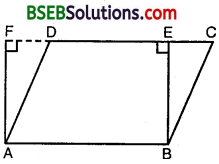To prove : Perimeter of ||gm ABCD > Perimeter of rectangle ABEF.
Proof : Since opposite sides of a ||gm and rectangle are equal.
∴ AB = DC [∵ ABCD is a ||gm]
AB = EF [∵ ABEF is a rectangle]
∴ DC = EF … (1)
⇒ AB + DC = AB + EF … (2)
Since, of all the segments that can be drawn to a given line from a point not lying on it, the perpendicular segment is the shortest.
∴ BE < BC and AF < AD ⇒ BC > BE and AD > AF
⇒ BC + AD > BE + AF … (3)
Adding (2) and (3), we get
AB + DC + BC + AD > AB + EF + BE + AF
⇒ AB + BC + CD + DA > AB + BE + EF + FA
Hence, perimeter of ||gm ABCD > perimeter of rectangle ABEF.Question 2.
In figure, D and E are two points on BC such that BD = DE = EC. Show that ar(ABD) = ar(ADE) = ar(AEC).Can you now answer the question that you have left in the ‘Introduction’, of this chapter, whether the field of Budhia has been actually divided into three parts of equal area?
Solution:
Let AL be perpendicular to BC. So, AL is the height of ∆s ABD, ADE and AEC.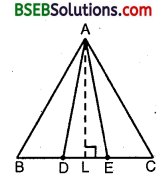∴ ar(ABD) = $$\frac { 1 }{ 2 }$$ x BD x AL
ar(ADE) = $$\frac { 1 }{ 2 }$$ x DE x AL
and, ar(AEC) = $$\frac { 1 }{ 2 }$$ x EC x AL
Since BD = DE = EC
∴ ar(ABD) = ar(ADE) = ar(AEC).
Yes, altitudes of all triangles are same. Budhia has use the result of this question in dividing her land in three equal parts.Question 3.
In figure, ABCD, DCFE and ABFE are parallelograms. Show that ar(ADE) = ar(BCF).Solution:
Since opposite sides of a ||gm are equal.
∴ AD = BC [∵ ABCD is a ||gm]
DE = CF [∵ DCFE is a ||gm]
and, AE = BF [∵ ABFE is a ||gm]
Consider As ADE and BCF, in which AE = BF, AD = BC and DE = CF
∴ By SSS criterion Of congruence

Question 4.
In figure, ABCD is a parallelogram and BC is produced to a point Q such that AD = CQ. If AQ intersect DC at P, show that ar(BPC) = ar(DPQ).Solution:
Join AC.
Since ∆s APC and BPC are on the same base PC and between the same parallels PC and AB.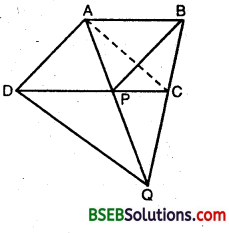∴ ar(APC) = ar(BPC) … (1)
∴ In the quadrilateral ADQC, one pair of opposite sides is equal and parallel.
⇒ AP = PQ and CP = DP [∵ Diagonals of a l|gm bisect each other]
In ∆s APC and DPQ, we have
AP = PQ [Proved above]
∠APC = ∠DPQ [Vertically opp.∠s]
and, PC = PD [Proved above]
∴ By SAS criterion of congruence,
∆ APC ≅ ∆ DPQ
⇒ ar(APC) = ar(DPQ) [∵ Congruent As have equal area]
∴ ar(BPC) = ar(DPQ).Question 5.
In figure, ABC and BDE are two equilateral triangles such that D is the mid-point of BC. If AE intersects BC at F, show that(i) ar(BDE) = $$\frac { 1 }{ 4 }$$ar(ABC)
(ii) ar(BDE) = $$\frac { 1 }{ 2 }$$ ar(BAE)
(iii) ar(ABC) = 2 ar(BEC)
(iv) ar(BFE) = ar(AFD)
(v) ar(BFE) = 2 ar(FED)
(vi) ar(FED) = $$\frac { 1 }{ 8 }$$ ar(AFC).
Solution:
Join EC and AD. Let a be the side of ∆ ABC. Then,(ii) We have, ar(BDE) = $$\frac { 1 }{ 2 }$$ ar(BEC) … (1) [∵ DE is a median of ∆ BEC and each median divides a triangle in two other As of equal area]
Now, ∠EBC = 60°
and ∠BCA = 60°
⇒ ∠EBC = ∠BCA.
But these are alternate angles with respect to the line- segments BE and CA and their transversal BC.
Hence, BE || AC.
Now, As BEC and BAE stand on the same base BE and lie between the same parallels BE and AC.
∴ ar(BEC) = ar(BAE)
∴ From (1), ar(∆ BDE) = $$\frac { 1 }{ 2 }$$ ar(BAE).

(iii) Since ED is a median of A BEC and we know that each median divides a triangle in two other As of equal area.
∴ ar(BDE) = $$\frac { 1 }{ 2 }$$ ar(BEC)
From part (i),ar(BDE) = $$\frac { 1 }{ 4 }$$ ar(ABC)
Combining these results, we get
$$\frac { 1 }{ 4 }$$ ar(ABC) = $$\frac { 1 }{ 4 }$$ ar(BEC)
⇒ ar(ABC) = 2ar(BEC).

(iv) Now, ∠ABD = ∠BDE = 60° (given)
But ∠ABD and ∠BDE are alternate angles with respect to the line-segment BA and DE and their transversal BD. Hence BA || ED.
Now, As BDE and AED stand on the same base ED and lie between the same parallels BA and DE
∴ ar (BDE) = ar(AED)
⇒ ar(BDE) – ar (FED) = ar(AED) – ar(FED)
⇒ ar(BEF) = ar (AFD)From (1) and (2), we have ar (AFD) = 2 ar (EFD)
Combining this result with part (iv).
We bave ar (BFE) = ar (AFD) = 2ar (EFD)
(vi) From part (i)Question 6.
Diagonals AC and BD of a quadrilateral ABCD intersect each other at P. Show that ar(APB) x ar(CPD) = ar(APD) x ar(BPC).
Solution:
Diagonals AC and BD of a quadrilateral ABCD intersect each other at P.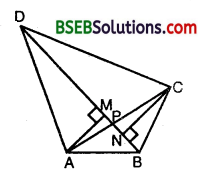Draw AM ⊥ BD and CN ⊥ BD.
Now, ar(APB) x ar(CPD)
= ($$\frac { 1 }{ 2 }$$ x BP x AM) x ($$\frac { 1 }{ 2 }$$ x DP x CN)
= $$\frac { 1 }{ 4 }$$ x BP x DP x AM x CN … (1)
and, ar(APD) x ar(BPC)
= ( $$\frac { 1 }{ 2 }$$ x DP x AM) x ($$\frac { 1 }{ 2 }$$ x BP x CN)
= $$\frac { 1 }{ 4 }$$ x BP x DP x AM x CN … (2)
From (1) and (2), we have
ar(APB) x ar(CPD) = ar(APD) x ar(BPC).Question 7.
P and Q are respectively the mid-points of sides AB and BC of a triangle ABC and R is the mid-point of AP, show that
(i) ar(PRQ) = $$\frac { 1 }{ 2 }$$ ar(ARC)
(ii) ar(RQC) = $$\frac { 3 }{ 8 }$$ ar(ABC)
(iii) ar(PBQ) = ar(ARC).
Solution:
P and Q are respectively the npd-points of sides AB and BC of Δ ABC and R is the mid-point of AP.
Join AQ and PC.(i) We have,
ar(PQR) = $$\frac { 1 }{ 2 }$$ ar(APQ) [∵ QR is a median of ∆ APQ and it divides the ∆ into two other ∆s of equal area]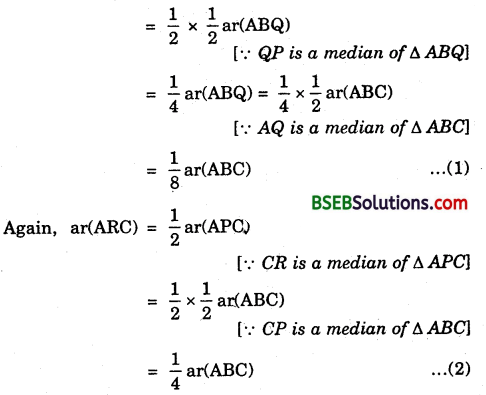From (1) and (2), we get .
ar(PQR) = $$\frac { 1 }{ 8 }$$ ar(ABC) = $$\frac { 1 }{ 2 }$$ x $$\frac { 1 }{ 4 }$$ ar(ABC)
= $$\frac { 1 }{ 2 }$$ ar(ARC).

(ii) We have,From (3), (4), (5) and (6), we have(iii) We have,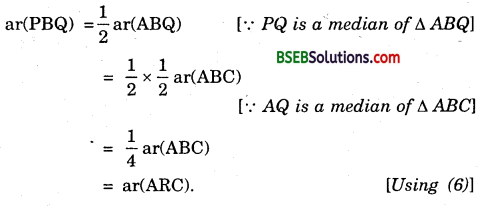Question 8.
In figure, ABC is a right triangle right angled at A. BCED, ACFG and ABMN are squares on the sides BC, CA and AB respectively. Line segment AX ⊥ DE meets BC at Y. Show that :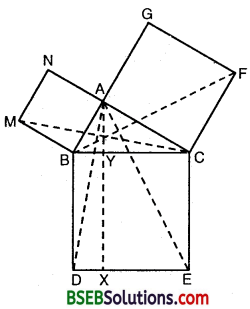(i) ∆ MBC ≅ ∆ ABD
(ii) ar(BYXD) = 2 ar(MBC)
(iii) ar(BYXD) = ar(ABMN)
(iv) ∆ FCB ≅ ∆ ACE
(vi) ar(CYXE) = ar(ACFG)
(vii) ar(BCED) = ar(ABMN) + ar(ACFG)
Note : Result (vii) is the famous Theorem of Pythagoras. You shall learn a simpler proof of this theorem in Class X.
Solution:
(i) In As MBC and ABD, we have
BC = BD [Sides of the square BCED]
MB = AB [Sides of the square ABMN]
∠MBC = ∠ABD [∵ Each = 90° + ∠ABC]
By SAS criterion of congruence, we have
∆ MBC ≅ ∆ ABD.

(ii) ∆ ABD and square BYXD have the same base BD and are between the same parallels BD and AX.
∴ ar(ABD) = $$\frac { 1 }{ 2 }$$ar(BYXD)
But ∆ MBC ≅ ∆ ABD [Proved in part (i)]
⇒ ar(MBC) = ar(ABD)
∴ ar(MBC) = ar(ABD) = $$\frac { 1 }{ 2 }$$ ar(BYXD)
⇒ ar(BYXD) = 2 ar(MBC).

(iii) Square ABMN and ∆ MBC have the same base MB and are between same parallels MB and NAC.
ar(MBC) = $$\frac { 1 }{ 2 }$$ ar(ABMN)
⇒ ar(ABMN) = 2ar(MBC)
= ar(BYXD) [Using part (ii)]

(iv) In ∆s ACE and BCF, we have
CE = BC [Sides of the square BCED]
AC = CF [Sides of the square ACFG]
and, ∠ACE = ∠BCF [∵ Each = 90° + ∠BCA]
∴ By SAS criterion of congruence,
∆ ACE ≅ ∆ BCF.

(v) ∆ ACE and square CYXE have the same base CE and are between same parallels CE and AYX.
∴ ar(ACE) = $$\frac { 1 }{ 2 }$$ ar(CYXE)
⇒ ar(FCB) = $$\frac { 1 }{ 2 }$$ ar(CYXE)[∵ ∆ ACE ≅ ∆ BCF, part (iv)]
⇒ ar(CYXE) = 2 ar(FCB).

(vi) Square ACFG and A BCF have the same base CF and are between same parallels CF and BAG.
∴ ar(BCF) = $$\frac { 1 }{ 2 }$$ar(ACFG)
⇒ $$\frac { 1 }{ 2 }$$ ar(CYXE) = $$\frac { 1 }{ 2 }$$ar(ACFG) [Using part (v)]
ar(CyXE) = ar(ACFG)

(vii) From part (Hi) and (vi), we have ar(BYXD) = ar(ABMN)
and,ar( CYXE) = ar(ACFG)